Updated: 4/5/2022

# Statistical Measures

0%
Topic
Review Topic
0
0
N/A
N/A
Questions
14
0
0
0%
0%
Evidence
4
0
0
Topic
 Standard Deviation vs. Standard Error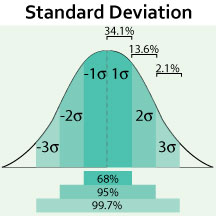n = sample size Sigma (σ) = standard deviation SEM = standard error of the mean SEM = σ/√n SEM < σ SEM decreases as n increases z-scores 1 = +/- 1 σ around mean 2 = +/- 2 σ around mean 3 = +/- 3 σ around mean
 Confidence Interval (CI) Describes the range in which the mean would be expected to fall if the study were performed again and again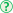= range from [mean - Z(SEM)] to [mean + Z(SEM)]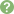95% CI (alpha = 0.05) is standard  for 95% CI, Z = 1.96 if 0 falls within the CI when calculating the difference between 2 variables, H0 is not rejected and the result is not significant if 1 falls within the CI when calculating OR or RR, H0 is not rejected and the result is not significant statistical significance refers to whether p < 0.05 clinical significance requires that a statistically significant result be also clinically meaningful T-test vs. ANOVA vs. χ2 T-testcompares the means of 2 groups on a continuous variable   ANOVA (analysis of variance)compares the means of 3 or more groups on a continuous variableχ2 ("chi-squared")tests whether 2 nominal variables are associatedused with 2x2 tables e.g., effect of treatment on disease Correlation Coefficient (r) Pearson coefficient, r, is always between -1 and +1 Absolute value indicates strength of correlation between 2 variablesCoefficient of determination = r2 Attributable Risk (AR)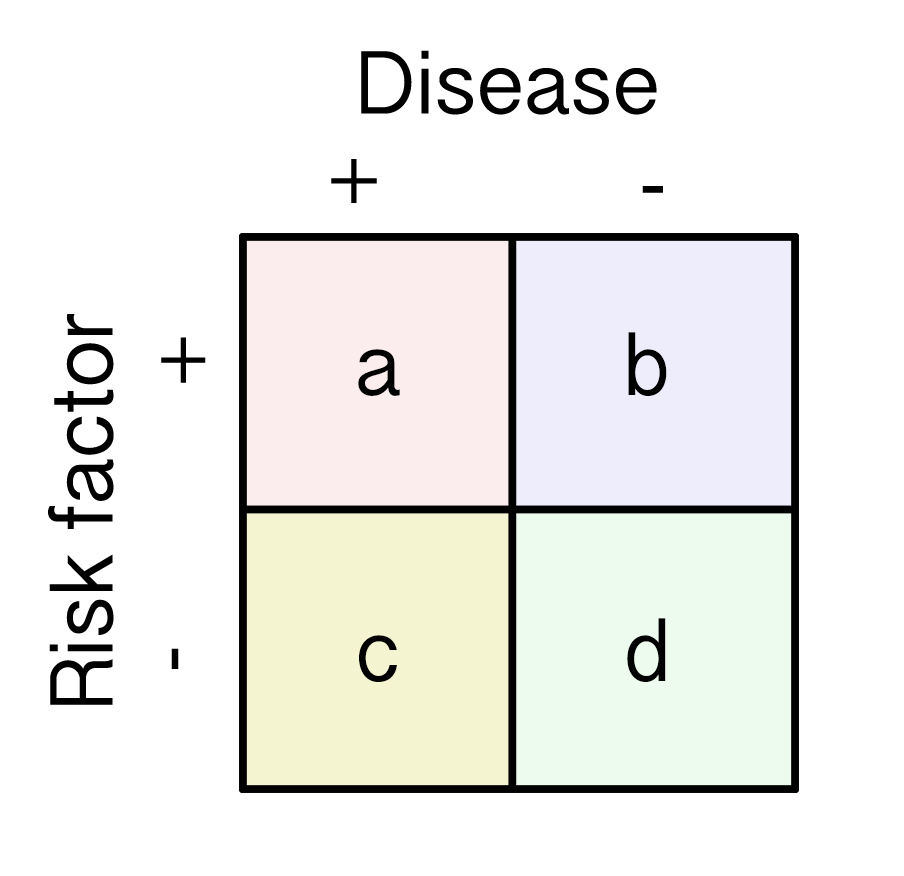AR is incidence in the exposed (Ie) - incidence in the unexposed (Iu) = Ie - Iu  Ie = a/(a+b) Iu = c/(c+d) AR = a/(a+b) - c/(c+d) The AR percent (ARP) is the attributable risk divided by incidence in the exposed (Ie)ARP = 100* (Ie-Iu)/Ie = 100*[a/(a+b) - c/(c+d)]/[a/(a+b)]   note that relative risk (RR) = Ie/Iu = a/(a+b) DIVIDED BY c/(c+d) using math tricks ARP = (RR-1)/RR

Average 3.8 of 19 Ratings

Questions (14)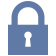Sorry, this question is forSorry, this question is forSorry, this question is forSorry, this question is forSorry, this question is for

(M1.ST.14.71) A study comparing the efficacy of four different weight loss diets is conducted. The primary outcome is change in weight (in kilograms) after two years. Prior to the study, there was insufficient evidence to conclude that any of the diets were effective. Figure A reports the mean weight change and the 95% confidence intervals (CI) for each of the four diets. Which of the following diets would have a p value < 0.05?

QID: 103888
FIGURES:
1

Atkins diet

25%

(17/69)

2

Low fat diet

20%

(14/69)

3

Low carbohydrate diet

6%

(4/69)

4

Zone diet

4%

(3/69)

5

Both the Atkins and the low fat diets

32%

(22/69)

M 1 D

Select Answer to see Preferred Response

(M1.ST.14.62) A prospective cohort study was conducted to assess the relationship between LDL and the incidence of heart disease. The patients were selected at random. Results showed a 10-year relative risk (RR) of 3.0 for people with elevated LDL levels compared to individuals with normal LDL levels. The p-value was 0.04 with a 95% confidence interval of 2.0-4.0. According to the study results, what percent of heart disease in these patients can be attributed to elevated LDL?

QID: 106444
1

25%

4%

(9/204)

2

33%

20%

(40/204)

3

50%

11%

(22/204)

4

67%

49%

(100/204)

5

100%

10%

(21/204)

M 1 E

Select Answer to see Preferred Response

(M1.ST.14.62) A prospective cohort study was conducted to assess the relationship between LDL-C and the incidence of heart disease. The patients were selected at random. Results showed a 10-year relative risk (RR) of 2.30 for people with elevated LDL-C levels compared to individuals with normal LDL levels. The p value was 0.04. This study is most likely to have which of the following 95% confidence intervals?

QID: 106440
1

1.01-3.70

45%

(31/69)

2

1.00-3.60

20%

(14/69)

3

0.09-3.50

10%

(7/69)

4

0.08-3.40

9%

(6/69)

5

0.07-3.30

3%

(2/69)

M 1 D

Select Answer to see Preferred ResponseSorry, this question is for

(M1.ST.14.76) You are conducting a systematic review on the effect of a new sulfonylurea for the treatment of type II diabetes. For your systematic review you would like to include 95% confidence intervals for the mean of blood glucose levels in the treatment groups. What further information is necessary to abstract from each of the original papers in order to calculate a 95% confidence interval for each study?

QID: 103893
1

Power, standard deviation, mean

9%

(7/81)

2

Power, mean, sample size

1%

(1/81)

3

Power, standard deviation, sample size

15%

(12/81)

4

Standard deviation, mean, sample size

53%

(43/81)

5

Standard deviation, mean, sample size, power

15%

(12/81)

M 1 E

Select Answer to see Preferred Response

(M1.ST.13.70) Confident of your performance on Step 1 given your extensive utilization of Medbullets, you preemptively start to ponder your future specialty choice. You come across an analysis of lifestyle factors and how they affect medical student specialty choices. Image A depicts two scatter plots comparing the relationship between median income and weekly work hours on the difficulty of matching into specific specialties. Both associations are statistically significant. Which statement best describes the results?

QID: 103887
FIGURES:
1

The harder the specialty is to match into, the higher the weekly work hours

0%

(0/52)

2

The easier the specialty is to match into, the higher the median income

0%

(0/52)

3

Weekly work hours has a stronger correlation with matching difficulty than median income

6%

(3/52)

4

Median income has a stronger correlation with matching difficulty than weekly work hours

85%

(44/52)

5

The higher the median income of a specialty the shorter the weekly work hours

6%

(3/52)

M 1 E

Select Answer to see Preferred Response

(M1.ST.13.78) An investigator is measuring the blood calcium level in a sample of female cross country runners and a control group of sedentary females. If she would like to compare the means of the two groups, which statistical test should she use?

QID: 103895
1

t-test

84%

(31/37)

2

ANOVA (Analysis of Variance)

5%

(2/37)

3

Linear regression

0%

(0/37)

4

Chi-square test

5%

(2/37)

5

F-test

0%

(0/37)

M 1 E

Select Answer to see Preferred Response

(M1.ST.13.74) A cross-sectional study is conducted investigating the effects of low salt diet on blood pressure. Five hundred patients identified as having a low salt diet and 500 patients identified as having a normal (control) diet were enrolled. All participants have their systolic blood pressure measured and the results are presented in Figure A. If the distribution of systolic blood pressure in each group is normal, what is the most appropriate test to determine whether patients on low salt diet have different systolic blood pressure than those on control diet?

QID: 103891
FIGURES:
1

Analysis of variance (ANOVA)

8%

(3/40)

2

One-sample t-test

10%

(4/40)

3

Two-sample t-test

52%

(21/40)

4

Paired t-test

12%

(5/40)

5

Chi Square test

12%

(5/40)

M 1 E

Select Answer to see Preferred ResponseSorry, this question is for